# Hand Gesture Classification using Deep Learning with Keras

• Ripul Agrawal
• Dec 17, 2019
• Deep LearningIn recent years, Deep Learning has become extremely popular, being the part of Machine Learning, it has several applications in today’s world from classifying pictures and translating languages to putting together a self-driving car. Today all these tasks are being controlled by computers itself with none human intervention.

Why Deep Learning?

In this “Big Data Era” of technology, we have a lot of data, which is not possible to process using traditional machine learning algorithms but possible with deep learning. It is obvious that if the data increase then deep learning will certainly increase the performance of the model. So Deep Learning is gaining much popularity due to its supremacy in terms of accuracy once trained with a vast amount of data.

Generally, deep learning is a machine learning algorithm in which we feed an input X and use it to predict an output Y like given some labeled images of cats and dogs and then use deep learning to make predictions for the new unlabelled image of cat or dog. Now the question arises is how deep learning does this? So basically deep learning algorithm is made up of a neural network that takes a labeled dataset and tries to find out the pattern between input and its labels like the human brain does and then based on this experience it makes predictions.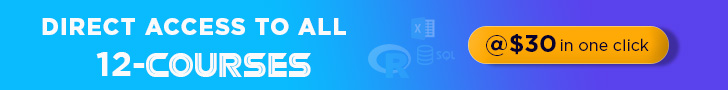It consists of an input layer, hidden layers and output layer which is made up of a collection of neurons (or nodes). Input layers take an array of numbers(i.e. In case of images -pixel values), predictions at the output layer, while hidden layers are correlated with most of the computation. When there is a case of a large number of neural layers in any network then it will be deep neural network and then only deep learning comes into existence, thus deep neural network improves its ability to approximate additional complex functions.

Types of Neural Network

There exist various types of neural networks for different tasks like Dense Neural Networks, Convolutional Neural Networks, Recurrent Neural Networks and many more. Apart from these layers, there exist other layers which include Pooling Layers, Dropout Layers, Activation Functions, etc, all these added up to make the outcomes of model efficient.

Convolutional Neural Network (CNN)

CNN is mainly used for computer vision which includes image classification, face recognition, object detection, etc. CNN, for image classification, followed by neural layers and activation functions takes an input image and treats it as an array of pixels, processes it by finding patterns in the image and then classifies it into certain categories or classes given in the problem statement. Image( the input layer) accepts will be either an array of RGB matrix ( 3 channels) or grayscale matrix (1 channel).

CNN Architecture

In deep learning, the CNN architecture will be composed of convolutional layers(filters), Pooling layers, fully connected layers (Dense) and then output layers.

Convolutional Layer

In this, the filter will be used to learn features from the image by convolving it with image, a mathematical operation, and then a new matrix will form i.e. with the learned features like edge detections, sharpen or blurred image.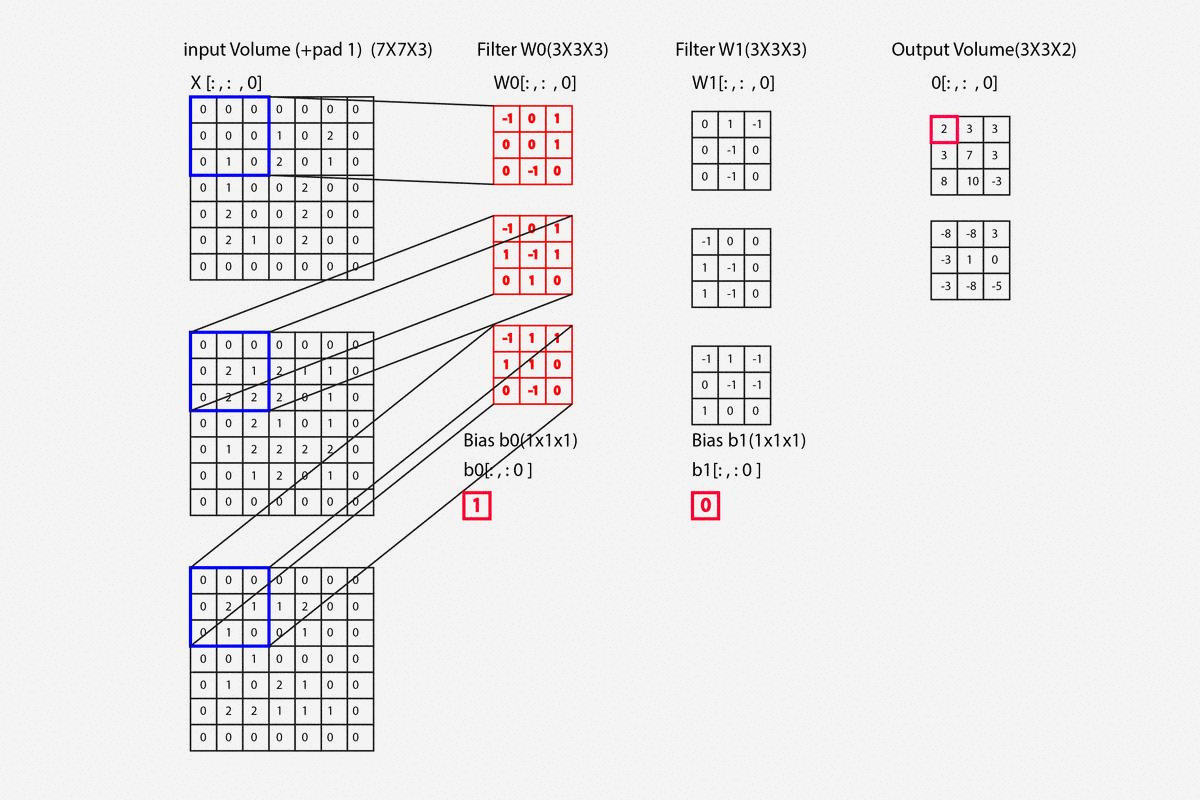Matrix visualization in a convolutional neural network

Pooling Layer

This layer would be used to perform downsampling operation on the input of this layer so that the input image will be compressed i.e. from a more detailed image to a less detailed image with more information. It can be of different types:

1. Max Pooling

2. Average Pooling

3. Sum Pooling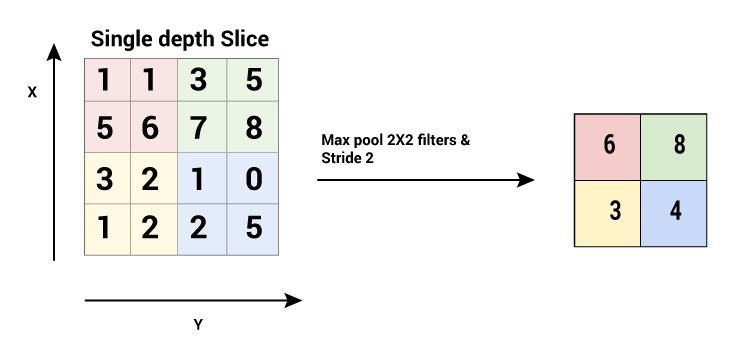Pooling layer in CNN architecture

Max Pooling takes the largest element from the rectified feature map and Sum Pooling takes the sum of all elements in the feature map.Fully Connected Layer

At this layer, just after the CNN network followed by pooling, the matrix will be flattened before feeding to Fully Connected Layer like a neural network. Then at the final layer, there will be activation function softmax or sigmoid to classify the output with the no of neurons in the final layer equals to no of classes in the output.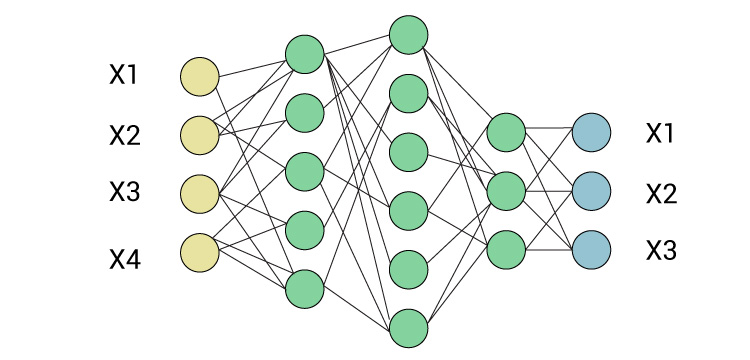Thread of fully connected layer between input and output data

Activation Function

It is a nonlinear layer put at the end of or in between neural networks which then decide the output of that node. Available activation functions are: Sigmoid, ReLU, Leaky ReLU, Softmax etc.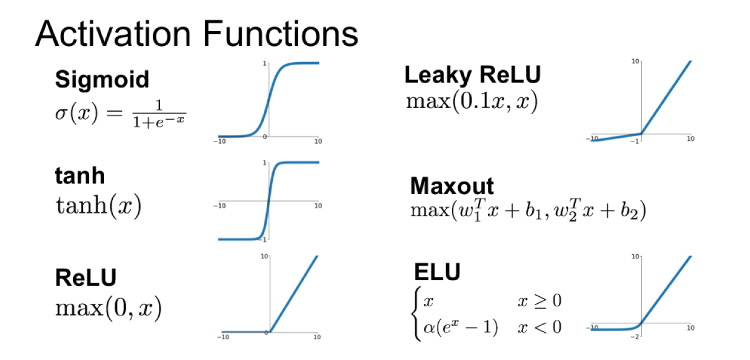List of various Activation Function

Hand Gesture Classification using Python Code

In the Hand Gesture Classification, we used a dataset that contains images of different hand gestures, such as a fist, palm, showing the thumb, and others which can be further useful to show counts from 1 to 5 with these hand gestures.

There are many algorithms in machine learning for classification out of which we'll be using Deep learning with the help of Convolution Neural Network (CNN) as discussed above, with the help of Keras ( an open-source neural network library written in Python). Keras is easy and fast and also provides support for CNN and runs seamlessly on both CPU and GPU.

Technologies Used:

1. Python3

2. Tools & Libraries used:

Import all the required libraries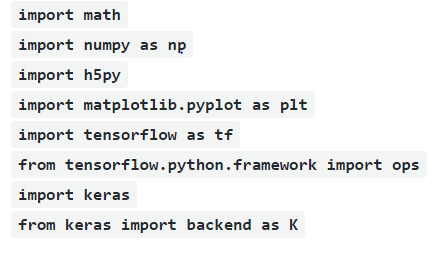We have two datasets one is the training dataset and the other is the test dataset. Training dataset containing 1080 images and test dataset containing 120 images with 6 labels to classify i.e. 0 to 5. Each image will be 64x64 pixels.

The dataset has been stored in the h5py format. The h5py package is a Pythonic interface for the HDF5 binary data format. With the help of HDF5, thousands of datasets can be stored in a single file. They support standard modes like r/w/a, so we'll load our dataset which contains images in r mode.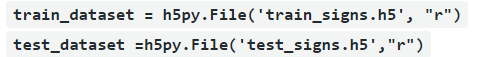After loading the dataset next step will be to get the image pixel's values in to set of arrays and labels as in different array for both training and test dataset as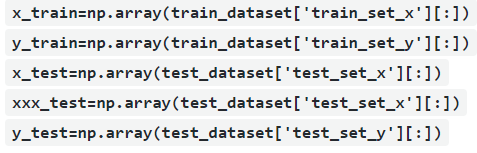Normalization of dataset Pixel values of the images will be from 0 to 255, so we'll normalize the dataset by dividing pixel values by 255.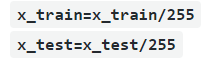converting class values to binary class metric for training and test set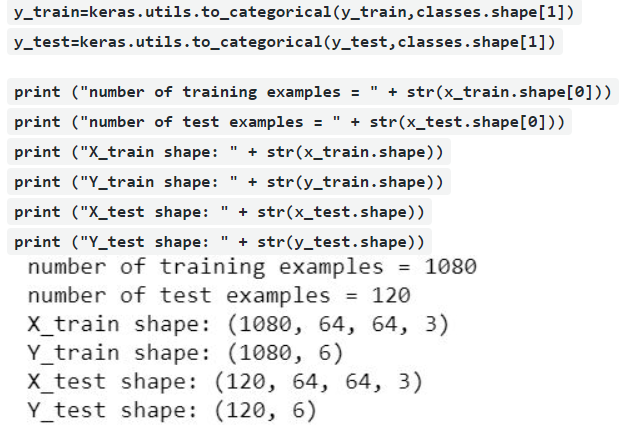Create CNN architecture using Keras

Sequential: It is a linear stack of layers, used for creating deep learning models like the instance of a Sequential model is created and layers will then be added to it piecewise.

Conv2D: This 2D convolution layer creates a kernel basically a filter that is convolved with the input layer (at the initial level with the image) and then produces a new array(tensor). There are some arguments we have to add while creating this layer are: filters (number of output filters in the convolution), kernel_size(dimension of the kernel), strides(an integer or tuple specifying the strides of the convolution along with height and width).

MaxPooling2D: This layer performs downsampling operation on the input of this layer which means it will compress the input image with more details to less detailed images as some features (like boundaries) have already identified, Conv2D it also accepts some arguments: pool_size(size of max pooling windows), strides, padding, etc.

Flatten: This flattens layer which flattens the inputs, will be added after the series of convolutional and pooling layers and followed by a fully connected dense layer which results in an N-dimensional vector, where N is the number of classes from which the model selects the desired class.

Dropout: It applies Dropout to the input. This is a technique used to prevent the model from overfitting by making weights of some redundant neurons in a particular layer equal to zero.

Import all the modules from Keras to create a CNN architecture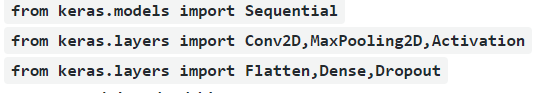Creates a model and adds layers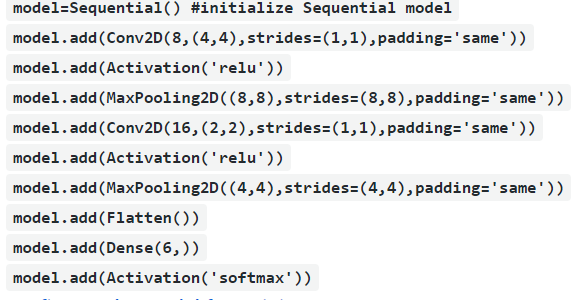As seen from code, we have created a neural network with 2 convolution layer followed by max pool layer with activation function “relu” in both layer, where the first layer has 8 filters of size (4,4) and size of max pool is (8,8) while the second layer has 16 filters of size (2,2) and size of max pool is (4,4), padding will be “same” in each layer. After the convolution layer, we’ll flatten the output of the convolution layer and then will add another fully connected layer(Dense) with 6 nodes in that because in our output we have 6 classes to predict.

Configures the model for training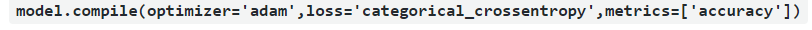Training of Model

We’ll train our model by feeding the labeled dataset (as loaded above) i.e. training dataset will be the training dataset and testing dataset will behave as the validation dataset. With the help of Keras, it will be easy to train our model using fit method and during the training, it will run off 140 iterations so as to update its weights and bias of the neural network with the help of optimizers,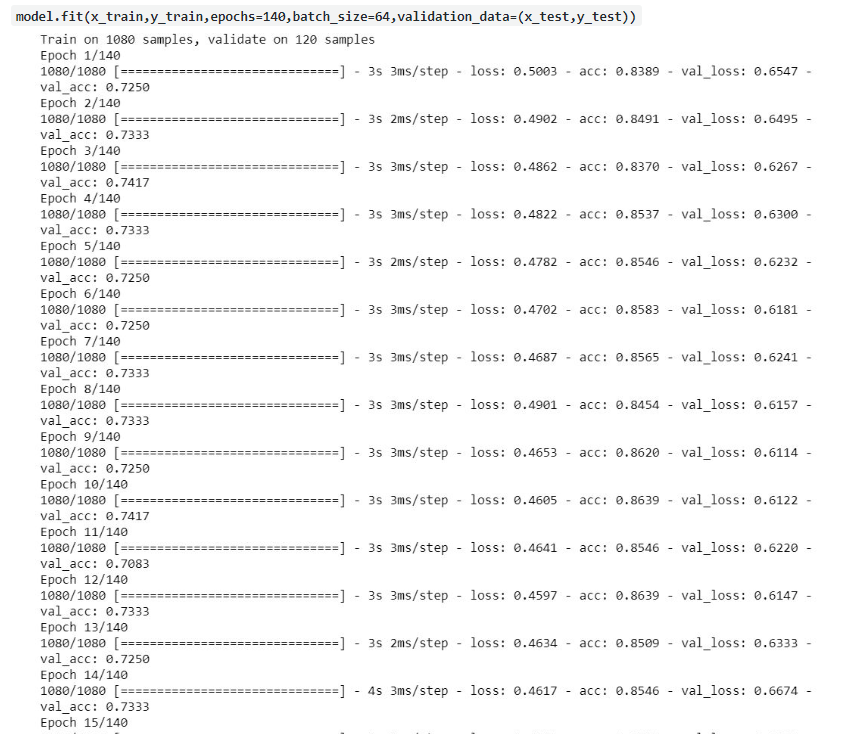Testing of the model

After the training of the model, we'll test the model that how accurate it will be on the new dataset i.e. test dataset using an evaluation method which returns loss value & accuracy value for the model in test mode, its input will be input test images and target variables i.e. x_test, y_test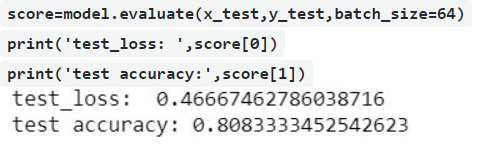Then we'll predict the output for the input samples i.e. test datasets using predict method.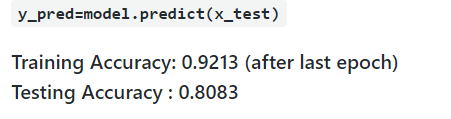Conclusion

Hand Gesture dataset has been classified using Convolution Neural Network with the help of Keras, an open-sourced neural-network library written in Python. Keras is preferred because it increases the computational power of deep neural network architecture by providing some functions that are needed for optimization like backpropagation, gradient descent, etc, and reduce the computation time. Keras has applications that provide some pre-trained models along with pre-trained weights which can be directly used for prediction, feature extraction and fine-tuning. For more updates and blogs on Analytics, Do read Analytics Steps.

0%